# RD Sharma Class 6 ex 20.4 Solutions Chapter 20 Mensuration

In this chapter, we provide RD Sharma Class 6 ex 20.4 Solutions Chapter 20 Mensuration for English medium students, Which will very helpful for every student in their exams. Students can download the latest RD Sharma Class 6 ex 20.4 Solutions Chapter 20 Mensuration Maths pdf, Now you will get step by step solution to each question.

# Chapter 20: Mensuration Exercise 20.4

### Question: 1

Question 1. Find the area of a rectangle, whose

(i) Length = 6cm, breadth = 3 cm

(ii) Length = 8 cm, breadth = 3 cm

(iii) Length = 4.5 cm, breadth = 2 cm

### Solution:

(i) Area of a rectangle = Length × Breadth

Length = 6 cm Breadth = 3 cm

Area of rectangle = 6 × 3 = 18 cm2

(ii) Area of a rectangle = Length × Breadth

Length = 8 cm Breadth = 3 cm

Area of rectangle = 8 × 3 = 24 cm2

(iii) Area of a rectangle = Length × Breadth

Length = 4.5 cm

Area of rectangle = 4.5 × 2 = 9 cm2

### Question: 2

Find the area of a square whose side is:

(i) 5 cm

(ii) 4.1 cm

(iii) 5.5 cm

(iv) 2.6 cm

### Solution:

Area of a square = Side × Side

(i) Side of the square = 5 cm

Area of the square = 5 × 5 = 25 cm2

(ii) Side of the square = 4.1 cm

Area of the square = 4.1 × 4.1 = 16.81 cm2

(iii) Side of the square = 5.5 cm

Area of the square = 5.5 × 5.5 = 30.25 cm2

(iv) Side of the square = 2.6 cm

Area of the square = 2.6 × 2.6 = 6.76 cm2

### Question: 3

The area of a rectangle is 49 cm2 and its breadth is 2.8 cm. Find the length of the rectangle.

### Solution:

Area = 49 cm2 Breadth = 2.8 cm

Area of the rectangle = Length × Breadth

= 49/2.8 = 17.5 cm

### Question: 4

The side of a square is 70 cm. Find its area and perimeter.

### Solution:

Side of the square = 70 cm

Area of the square = Side × Side = 70 × 70 = 4900 cm2

Perimeter of the square = 4 × Side

= 4 × 70 = 280 cm

### Question: 5

The area of a rectangle is 225 cm2 and its one side is 25 cm, find its other side.

### Solution:

Area = 225 cm2

One of the sides = 25 cm

Area of the rectangle = Product of the lengths of its two side

Other side = Area/Side = 225/25 = 9 cm

### Question: 6

What will happen to the area of a rectangle if its

(i) Length and breadth are trebled (ii) Length is doubled and breadth is same

(iii) Length is doubled

### Solution:

(i) If the length and breadth of a rectangle are trebled.

Let the initial length and breadth be l and b, respectively.

Original area = l × b = lb

Now,

the length and breadth are trebled which means they become three times of their original value.

Therefore New length = 3l

New area = 3l × 3b = 9lb

Thus, the area of the rectangle will become 9 times that of its original area.

(ii) If the length is doubled and the breadth is same.

Let the initial length and breadth be l and b, respectively.

Original area = l × b = lb

Now, length is doubled and breadth remains same.

Therefore New length = 2l

New area = 2l × b = 2 lb

Thus, the area of the rectangle will become 2 times that of its original area.

(iii) If the Length is doubled and breadth is halved.

Let the initial length and breadth be land b, respectively.

Original area =l × b = lb

Now, length is doubled and breadth is halved.

Therefore New length = 2l

New area = 2 l × b / 2 = lb

New area is also lb.

This means that the areas remain the same.

### Question: 7

What will happen to the area of a square if its side is :

(i) Tripled

(ii) increased by half of it

### Solution:

(i) Let the original side of the square be s.

Original area = s x s = s2

If the side of a square is tripled, new side will be equal to 3s.

New area = 3s × 3s = 9s2

This means that the area becomes 9 times that of the original area.

(ii) Let the original side of the square be s.

Original area = s × s = s2

If the side of a square is increased by half of it, new side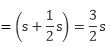This means that the area becomes 9/4 times that of the original area.

### Question: 8

Find the perimeter of a rectangle whose area is 500 cm2 and breadth is 20 cm.

### Solution:

Area = 500 cm2

Area of rectangle = Length × Breadth

= 500/20 = 25 cm

Perimeter of a rectangle = 2 (Length + Breadth)

= 2(25 + 20) cm = 2 × 45 cm = 90 cm

### Question: 9

A rectangle has the area equal to that of a square of side 80 cm. If the breadth of the rectangle is 20 cm,  Find its length.

### Solution:

Side of the square = 80 cm

Area of square = Side × Side = 80 × 80 = 6400 cm2

Given that:

Area of the rectangle = Area of the square = 6400 cm2

Breadth of the rectangle = 20 cm

Applying the formula:

Length of the rectangle = Area/Breadth

We get:

Length of the rectangle = 6400/20 = 320 cm

### Question: 10

Area of a rectangle of breadth 17 cm is 340 cm2. Find the perimeter of the rectangle.

### Solution:

Area of the rectangle = 340 cm2

Breadth of the rectangle = 17 cm

Applying the formula:

Length of a rectangle = Area / Breadth

We get:

Length of the rectangle = 340/17 = 20 cm

Perimeter of rectangle = 2 (Length + Breadth)

= 2 (20 + 17)

= 2 × 37

= 74 cm

### Question: 11

A marble tile measures 15 cm × 20cm. How many tiles will be required to cover a wall of size 4m × 6m?

### Solution:

Dimensions of the tile = 15 cm × 20 cm

Dimensions of the wall = 4 m × 6 m = 400 cm × 600 cm (Since, 1 m = 100 cm)

Area of the tile = 15 cm × 20 cm = 300 cm2

Area of the wall = 400 cm × 600 cm = 2, 40, 000 cm2

### Question: 12

A marble tile measures 10 cm × 12 cm. How many tiles will be required to cover a wall of size 3m × 4m? Also, find the total cost of the tiles at the rate of Rs 2 per tile.

### Solution:

Dimension of the tile = 10 cm × 12 cm

Dimension of the wall =3 m × 4 m = 300 cm × 400 cm (Since, 1 m = 100 cm)

Area of the tile = 10 cm × 12 cm = 120 cm2

Area of the wall = 300 cm × 400 cm = 1, 20, 000 cm2

Number of tiles required to cover the wall = Area of wall / Area of one tile

= 120000/120 = 1,000 tiles

Cost of tiles at the rate of Rs. 2 per tile = 2 × 1,000 = Rs. 2,000

### Question: 13

One tile of a square plot is 250 m, find the cost of leveling it at the rate of Rs 2 per square meter.

### Solution:

Side of the square plot = 250 m

Area of the square plot = Side × Side = 250 × 250 = 62,500 m2

Rate of leveling the plot = Rs. 2 per m2

Cost of leveling the square plot = Rs. 62,500 × 2 = Rs. 1, 25, 000

### Question: 14

The following figures have been split into rectangles. Find the areas. (The measures are given in centimeters)

### Solution: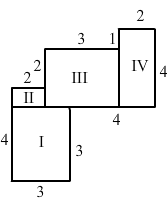(i) This figure consists of two rectangles II and IV and two squares I and III.

Area of square I = Side × Side = 3 × 3 = 9 cm=2

Similarity, area of rectangle II = (2 × 1) = 2 cm2

Area of square III = (3 × 3) = 9 cm2

Area of rectangle IV = (2 × 4) = 8 cm2

Thus, the total area of this figure = (Area of square I + Area of rectangle II + Area of square III + Area of rectangle IV) = 9 + 2 + 9 + 8 = 28 cm2

(ii) This figure consists of three rectangles I, II and Ill.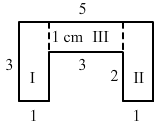Area of rectangle I = Length × Breadth = 3 × 1 = 3 cm2

Similarly, area of rectangle II = (3 × 1) = 3 cm2

Area of rectangle III = (3 × 1) = 3 cm2

Thus,

the total area of this figure = (Area of rectangle I + area of rectangle II + area of rectangle III)

= 3 + 3 + 3 = 9 cm2

### Question: 15

Split the following shapes into rectangles and find the area of each. (The measures are given in centimeters)

###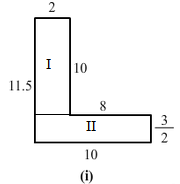Solution:

(i) This figure consists of two rectangles I and II.

The area of rectangle I = Length × Breadth = 10 × 2 = 20 cm2

Similarly, area of rectangle II = 10 × 32 = 15 cm2

Thus, total area of this figure = (Area of rectangle I + Area of rectangle II) = 20 + 15 = 35 cm2

(ii) This figure consists of two squares I and III and one rectangle II.

Area of square I = Area of square III = Side × Side = 7 × 7 = 49 cm2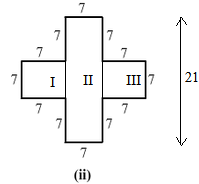Similarly, area of rectangle II = (21 × 7) = 147 cm2

Thus, total area of this figure = (Area of square I + Area of rectangle II + Area of square III)

= 49 + 49 + 147 = 245 cm2

(iii) This figure consists of two rectangles I and II.

Area of rectangle I = Length × Breadth = 5 × 1 = 5 cm2

Similarly, area of rectangle II = 4 × 1 = 4 cm2Thus, total area of this figure = (Area of rectangle I + Area of rectangle II) = 5 + 4 = 9 cm2

### Question: 16

How many tiles with dimension 5 cm and 12 cm will be needed to fit a region whose length and breadth are respectively?

(i) 100 cm and 144 cm

(ii) 70 cm and 36 cm

### Solution:

(i) Dimension of the tile = 5 cm × 12 cm

Dimension of the region = 100 cm × 144 cm

Area of the tile = 5 cm × 12 cm = 60 cm2

Area of the region = 100 cm × 144 cm = 14,400 cm2

Number of tiles required to cover the region = Area of the region / Area of one tile

= 14400/60 = 240 tiles

(ii) Dimension of the tile = 5 cm × 12 cm

Dimension of the region = 70 cm × 36 cm

Area of the tile = 5 cm × 12 cm = 60 cm2

Area of the region = 70 cm × 36 cm = 2,520 cm2

Number of tiles required to cover the region = Area of the region / Area of one tile

= 2520/42 = 42 tiles

All Chapter RD Sharma Solutions For Class 6 Maths

I think you got complete solutions for this chapter. If You have any queries regarding this chapter, please comment on the below section our subject teacher will answer you. We tried our best to give complete solutions so you got good